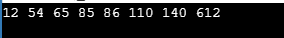# How To Best Implement Radix Sort Program In C?

Published on Sep 10,2019 2.6K ViewsSo let us get started then,

In simple words, sorting means arranging the given elements in a systematic order. Sorting is done in most of the algorithms because it makes search easier which eventually makes the algorithm efficient. In this blog we will understand one of the commonly used soring algorithms i.e. Radix sort.

Radix sort is a non-comparative integer sorting algorithm. It does digit by digit sorting starting from least significant digit(i.e. digit present at the right) to most significant digit(i.e. digit present at the left). Radix sort uses counting sort as a subroutine to sort.
The lower bound for Comparison based sorting algorithm (such as Heap sort, Quick Sort, Merge Sort) is Ω(nLogn), & they it can’t be improved beyond nLogn. If we talk about counting sort, it is a linear time sorting algorithm with O(n+k) time complexity, where the range lies between 1 to k. Now, the problem with counting sort is, it takes O(n2) when the elements range from 1 to n2.

So, to sort an array with elements that range from 1 to n2 in linear time, we need radix sort. Radix sort sorts the array digit by digit starting from least significant digit to most significant digit. Radix sort uses counting sort as a subroutine to sort.

Perform the following steps for all the digits starting from the least significant digit present in right, moving towards most significant digit present in left.

Sort the elements using counting sort according to the current digit.
Example:

Original Array:
140, 65, 85, 110, 612, 54, 12, 86

Sorting the least significant digit i.e. at one’s place, gives

140, 110, 612, 12, 54, 65, 85, 86

NOTE: As 612 appears before 12, and the sorting is done only for one’s digit, thus 612 appears before 12 after this iteration.

Sorting by next digit, i.e. at 10s place, gives:

110, 612, 12, 140, 54, 65, 85, 86

Sorting by most significant digit, i.e. present at the 100s place, gives:

012, 054, 065, 085, 086, 110, 140, 612

## Radix Sort Program In C

First look at Radix sort function

```void radixsort(int array[], int n) {
//Get the largest number to know the maximum number of digits
int m = getMax(array, n);
int dig;
//Counting sort is performed for every digit
for (dig = 1; m / dig > 0; dig *= 10)
countSort(array, n, dig);
}
```

## Count Sort Function:

```void countSort(int array[], int n, int dig) {
int output[n];
int i, count = { 0 };
// Store count of occurrences in count[]
for (i = 0; i < n; i++)
count[(array[i] / dig) % 10]++;
// Change count[i] so that count[i] now contains actual
//  position of this digit in output[]
for (i = 1; i < 10; i++) count[i] += count[i - 1]; // Build the output array for (i = n - 1; i >= 0; i--) {
output[count[(array[i] / dig) % 10] - 1] = array[i];
count[(array[i] / dig) % 10]--;
}
// Copy the output array to arr[], so that arr[] now
// contains sorted numbers according to current digit
for (i = 0; i < n; i++)
array[i] = output[i];
}
```

Example:

```#include<stdio.h>
//Function to find the Largest Number
int getMax(int array[], int n) {
int max = array;
int i;
for (i = 1; i < n; i++) if (array[i] > max)
max = array[i];
return max;
}
//Function for Count sort
void countSort(int array[], int n, int dig) {
int output[n];
int i, count = { 0 };
// Store count of occurrences in count[]
for (i = 0; i < n; i++)
count[(array[i] / dig) % 10]++;
// Change count[i] so that count[i] now contains actual
//  position of this digit in output[]
for (i = 1; i < 10; i++) count[i] += count[i - 1]; // Build the output array for (i = n - 1; i >= 0; i--) {
output[count[(array[i] / dig) % 10] - 1] = array[i];
count[(array[i] / dig) % 10]--;
}
// Copy the output array to arr[], so that arr[] now
// contains sorted numbers according to current digit
for (i = 0; i < n; i++) array[i] = output[i]; } // The main function to that sorts arr[] of size n using Radix Sort void radixsort(int array[], int n) { //Get the largest number to know the maximum number of digits int m = getMax(array, n); int dig; //Counting sort is performed for every digit for (dig = 1; m / dig > 0; dig *= 10)
countSort(array, n, dig);
}
//Function to Print Array
void print(int arr[], int n) {
int i;
for (i = 0; i < n; i++)
printf("%d ", arr[i]);
}
int main() {
int arr[] = { 140, 65, 85, 110, 612, 54, 12, 86 };
int n = sizeof(arr) / sizeof(arr);
print(arr, n);
return 0;
}
```

OutputNow after executing the above program you would have understood the Radix Sort Program In C. Thus we have come to an end of this article on ‘Quicksort in Java’. If you wish to learn more, check out the Java Training by Edureka, a trusted online learning company. Edureka’s Java J2EE and SOA training and certification course is designed to train you for both core and advanced Java concepts along with various Java frameworks like Hibernate & Spring.

Got a question for us? Please mention it in the comments section of this blog and we will get back to you as soon as possible.